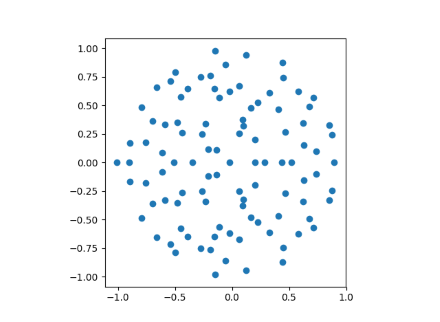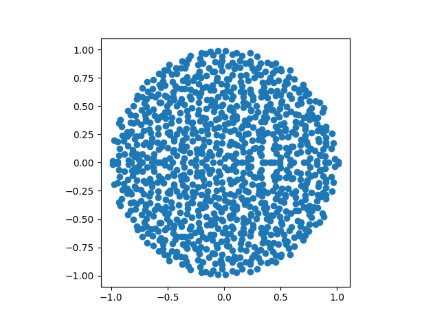# Circular law for random matrices

Suppose you create a large matrix M by filling its components with random values. If has size n by n, then we require the probability distribution for each entry to have mean 0 and variance 1/n. Then the Girko-Ginibri circular law says that the eigenvalues of M are approximately uniformly distributed in the unit disk in the complex plane. As the size n increases, the distribution converges to a uniform distribution on the unit disk.

The probability distribution need not be normal. It can be any distribution, shifted to have mean 0 and scaled to have variance 1/n, provided the tail of the distribution isn’t so thick that the variance doesn’t exist. If you don’t scale the variance to 1/n you still get a circle, just not a unit circle.

We’ll illustrate the circular law with a uniform distribution. The uniform distribution has mean 1/2 and variance 1/12, so we will subtract 1/2 and multiply each entry by √(12/n).

Here’s our Python code:

```    import matplotlib.pyplot as plt
import numpy as np

n = 100
M = np.random.random((n,n)) - 0.5
M *= (12/n)**0.5
w = np.linalg.eigvals(M)
plt.scatter(np.real(w), np.imag(w))
plt.axes().set_aspect(1)
plt.show()
```

When n=100 we get the following plot.When n=1000 we can see the disk filling in more.Note that the points are symmetric about the real axis. All the entries of M are real, so its characteristic polynomial has all real coefficients, and so its roots come in conjugate pairs. If we randomly generated complex entries for M we would not have such symmetry.

Related post: Fat-tailed random matrices

## 2 thoughts on “Circular law for random matrices”

1. Circular low is fundamental in deep learning via spectral ergodicity, i.e., dynamics of weight matrices.

2. atan2(x,y) of two standard normal’s gives back the uniform distribution.